## How to Compare and Order Numbers?

Practice Unlimited Questions

#### What is comparing numbers?

The process to find if a number is greater than, smaller than or equal to the other number is called number comparison.

#### What is the ordering of numbers?

The process of arranging given numbers either in increasing order (ascending order) or in decreasing order (descending order) is called ordering of numbers.

#### How do you compare four digit numbers?

We follow the below steps to find out which number is greater or smaller.

Step 1: Check the digits in Thousands place, the number which has a bigger digit is greater. If the two numbers have the same number of thousands then we compare the hundreds.

Step 2: Check the digits in Hundreds place, the number which has a bigger digit is greater. If they also have the same number of hundreds then we compare the tens.

Step 3: Check the digits in Tens place, the number which has a bigger digit is greater. If they also have the same number of tens then we compare the ones.

Step 4: Check the digits in Ones place, the number which has a bigger digit is greater.

#### Example of a comparison - Which number is greater: 2621 or 2683?

First, we create a place value chart for both these numbers as shown below.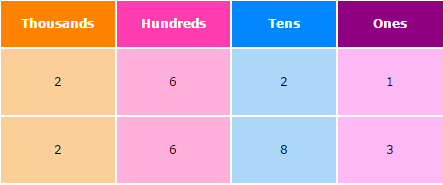Step 1: Check the digit in Thousands place. Both digits are same: 2

Step 2: Check the digit in Hundreds place. Both digits are same: 6

Step 3: Check the digit in Tens Place. 8 is greater than 2. Hence number 2683 is greater than 2621 or in other words 2621 is smaller than 2683.

#### 1. Joy has a collection of 3046 toy cars. Anil has a collection of 2158 toy cars. Who has a bigger collection of toy cars?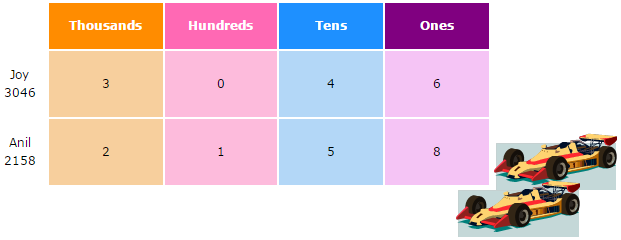Compare the thousands. 3 thousands is greater than 2 thousands.

So, 3026 is greater than 2158.

Joy has a bigger collection of toy cars.

#### 2. A florist had 2621 roses. He had 2683 orchids. Did he have fewer roses than orchids?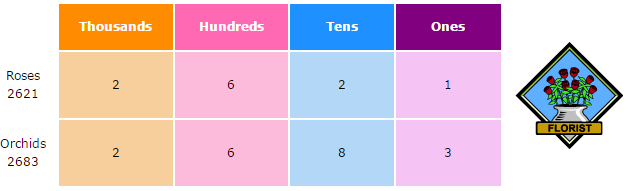If the two numbers have the same number of thousands then we compare the hundreds.

If they also have the same number of hundreds then we compare the tens.

If they also have the same number of tens then we compare the ones. First, compare the thousands. They are the same.

Then, compare the hundreds. They are also the same.

Next, compare the tens. 2 tens is smaller than 8 tens.

So, 2621 is smaller than 2683.

Yes, there were fewer roses than orchids.

#### 3. Compare the following numbers -> 4026, 2940 and 4377 a) Which number is the smallest? b) Which number is the greatest? c) Arrange the numbers in order, beginning with the smallest. d) Arrange the numbers in order, beginning with the greatest.

a)   Compare the thousands.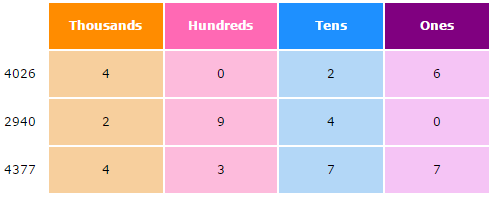2 thousands is smaller than 4 thousands.

So, 2940 is the smallest number.

b)   Compare the remaining two numbers.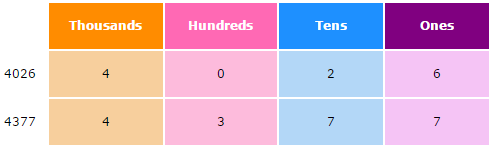First, compare the thousands. They are the same.

Next, compare the hundreds. 3 hundreds is greater than 0 hundreds.

So, 4377 is the greatest number.

c)   Arrange the three numbers in order, beginning with the smallest.

2940       4026       4377
4026 is greater than 2940 but smaller than 4377.

d)   Arrange the three numbers in order, beginning with the greatest.

4377       4026       2940

#### 4. Falaq has four balls as shown below.a) What is the biggest 4-digit number she can make with them? b) What is the smallest 4-digit number she can make with them?

a)   To get the biggest 4-digit number,
• The ball with the biggest value should be placed in the thousands place,
• The ball with the second biggest value should be placed in the hundreds place, and
• So on.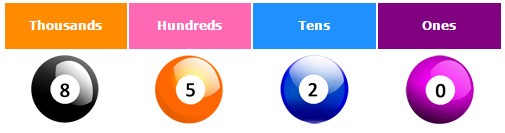The biggest 4-digit number she can make is 8520.

b)   To get the smallest 4-digit number,
• The ball with the smallest value should be placed in the thousands place,
• The ball with the second smallest value should be placed in the hundreds place, and
• So on.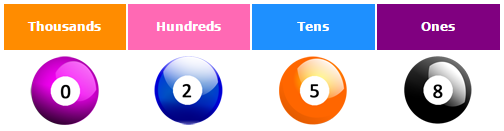The above number is a 3-digit number since 0 has no value if it is put in the highest value place i.e. the thousands place.

To get a 4-digit number, we switch the places of the digits in the thousands place and the hundreds place.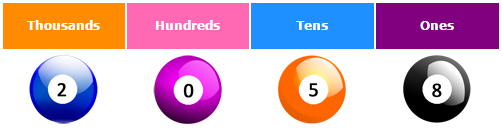The smallest 4-digit number she can make is 2058.

#### 5. Rayan has four balls as shown below.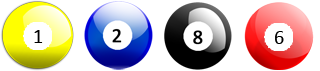a) What is the biggest 4-digit even number he can make with them? b) What is the smallest 4-digit odd number he can make with them?

a)   To get the biggest 4-digit even number, we should choose the ball with the smallest even value for the ones place: 2.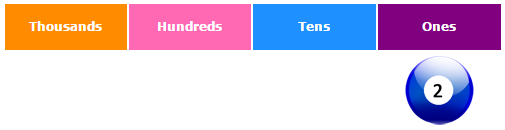Arrange the remaining balls (1, 8 and 6) as follows to get the biggest possible number:
• The ball with the biggest value in the thousands place,
• The ball with the second biggest value in the hundreds place, and
• So on.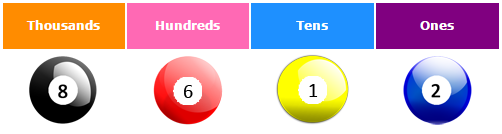The biggest 4-digit even number he can make is 8612.

b)   To get an odd number, the digit in the ones place should be odd: 1.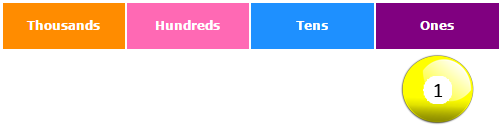Arrange the remaining balls (2, 8 and 6) as follows to get the smallest possible number:
• The ball with the smallest value in the thousands place,
• The ball with the second smallest value in the hundreds place, and
• So on.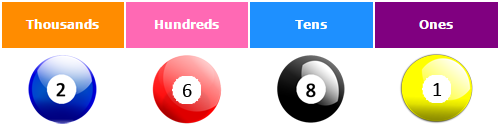The smallest 4-digit odd number he can make is 2681.